# node js - node js timer - node - node js tutorial - webnode

## What is Timer in Node.js?

• Node.js Timer functions are global functions.
• You don't need to use require() function in order to use timer functions.
• Let's see the list of timer functions.

## Set timer functions:

• setImmediate(): It is used to execute setImmediate.
• setInterval(): It is used to define a time interval.
• setTimeout(): ()- It is used to execute a one-time callback after delay milliseconds

## Clear timer functions:

• clearImmediate(immediateObject): It is used to stop an immediateObject, as created by setImmediate
• clearInterval(intervalObject): It is used to stop an intervalObject, as created by setInterval
• clearTimeout(timeoutObject): It prevents a timeoutObject, as created by setTimeoutLearn Node js - node js Tutorial - Nodejs Timer Image - node - Node js Examples

## Node.js Timer setInterval() Example

• This example will set a time interval of 1000 millisecond and the specified comment will be displayed after every 1000 millisecond until you terminate.

## Node JS Output :Learn Node js - node js Tutorial - Node.js Timer Example1 - node - Node js Examples

## Node JS Output :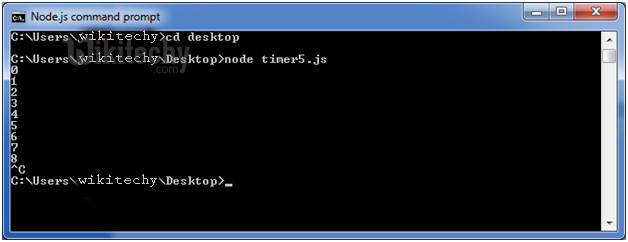Learn Node js - node js Tutorial - Node.js Timer Example2 - node - Node js Examples

## Node JS Output :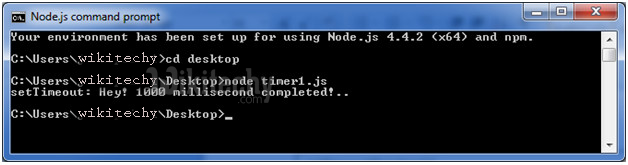Learn Node js - node js Tutorial - Node.js Timer Example3 - node - Node js Examples

• This example shows time out after every 1000 millisecond without setting a time interval. This example uses the recursion property of a function.

## Node JS Output :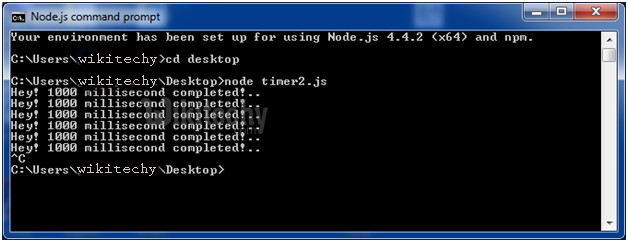Learn Node js - node js Tutorial - Node.js Timer Example5 - node - Node js Examples

## Node.js setInterval(), setTimeout() and clearTimeout()

• Let's see an example to use clearTimeout() function.

## Node JS Output :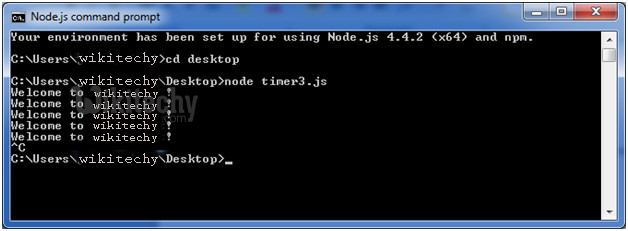Learn Node js - node js Tutorial - Node.js Timer Example5 - node - Node js Examples

• You can see that the above example is recursive in nature. It will terminate after one step if you use ClearInterval.

## Node.js setInterval(), setTimeout() and clearInterval()

• Let's see an example to use clearInterval() function.

## Node JS Output :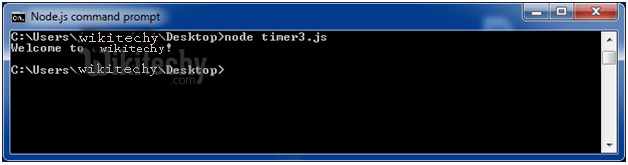Nodejs Timer Example6